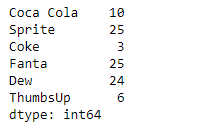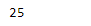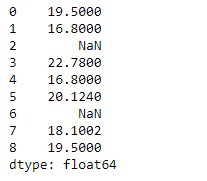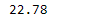# Python | Pandas Series.max()

Pandas series is a One-dimensional ndarray with axis labels. The labels need not be unique but must be a hashable type. The object supports both integer- and label-based indexing and provides a host of methods for performing operations involving the index.

Pandas` Series.max()` function return the maximum of the underlying data in the given Series object. This function always returns Series even if only one value is returned.

Syntax: Series.max(axis=None, skipna=None, level=None, numeric_only=None, **kwargs)

Parameter :
axis : Axis for the function to be applied on.
skipna : Exclude NA/null values when computing the result.
level : If the axis is a MultiIndex (hierarchical), count along a particular level, collapsing into a scalar.
numeric_only : Include only float, int, boolean columns.
**kwargs : Additional keyword arguments to be passed to the function.

Returns : max : scalar or Series (if level specified)

Example #1: Use `Series.max()` function to find the maximum value among the underlying data in the given series object.

 `# importing pandas as pd ` `import` `pandas as pd ` ` `  `# Creating the Series ` `sr ``=` `pd.Series([``10``, ``25``, ``3``, ``25``, ``24``, ``6``]) ` ` `  `# Create the Index ` `index_ ``=` `[``'Coca Cola'``, ``'Sprite'``, ``'Coke'``, ``'Fanta'``, ``'Dew'``, ``'ThumbsUp'``] ` ` `  `# set the index ` `sr.index ``=` `index_ ` ` `  `# Print the series ` `print``(sr) `

Output :Now we will use `Series.max()` function to find the maximum value of the given series object.

 `# return the maximum value in the  ` `# series object ` `result ``=` `sr.``max``() ` ` `  `# Print the result ` `print``(result) `

Output :As we can see in the output, the `Series.max()` function has successfully returned the maximum value of the given series object.

Example #2: Use `Series.max()` function to find the maximum value among the underlying data in the given series object. The given series object also contains some missing values.

 `# importing pandas as pd ` `import` `pandas as pd ` ` `  `# Creating the Series ` `sr ``=` `pd.Series([``19.5``, ``16.8``, ``None``, ``22.78``, ``16.8``, ``20.124``, ``None``, ``18.1002``, ``19.5``]) ` ` `  `# Print the series ` `print``(sr) `

Output :Now we will use `Series.max()` function to find the maximum value of the given series object. we are going to skip the missing value while finding the maximum value.

 `# return the maximum value in the series object ` `# skip the missing values ` `result ``=` `sr.``max``(skipna ``=` `True``) ` ` `  `# Print the result ` `print``(result) `

Output :As we can see in the output, the `Series.max()` function has successfully returned the maximum value of the given series object.

My Personal Notes arrow_drop_upCheck out this Author's contributed articles.

If you like GeeksforGeeks and would like to contribute, you can also write an article using contribute.geeksforgeeks.org or mail your article to contribute@geeksforgeeks.org. See your article appearing on the GeeksforGeeks main page and help other Geeks.

Please Improve this article if you find anything incorrect by clicking on the "Improve Article" button below.

Article Tags :

Be the First to upvote.

Please write to us at contribute@geeksforgeeks.org to report any issue with the above content.